# How to Change the Background of an Image with Green Screen Background in MATLAB?

• Difficulty Level : Expert
• Last Updated : 17 Dec, 2021

Have you ever think how graphics or VFX movies are made with such background scenes? Actually, all the VFX-designed movies are made using a green screen. The same concept we are going to discuss in this article. An image with a completely green background is called a green background image. Another image of the same size is being considered here. We shall add the background to the image which has a green background.

Green Screen or Chroma Key photography is a photography style in which we shoot pictures with a solid color in the background So that it becomes easy for the editors to replace that solid background with beautiful backgrounds. This kind of photography is mandatory for every motion picture that involves graphics.

It is not necessary to use green screen only. We have other options as well for the color of the screen. We can choose any color for the background screen, provided that the background color should not be present in any portion of the foreground subject image.

Steps:

• Read the green screen image.
• Loop over the green image, if a pixel is completely green then it means it belongs to the background and needs to be replaced. Then,
• replace that pixel with the corresponding pixel information from the background image.
• Display the images to illustrate the working of the concept.

Function Used:

• imtool( ) inbuilt function is used to display the image.
• size( ) inbuilt function is used to get size of the image.
• rgb2gray( ) inbuilt function is used to convert RGB image to Grayscale image.
• imresize( ) inbuilt function is used to resize the original image.
• length( ) inbuilt function is used to get length of the array.

Note: The size of the green screen image and background image must be equal in order to execute the code successfully in the desired manner.

If we use different sized images then the size of the final image will be equal to the size of the green screen image.

Both images should be RGB-colored images.

Example:

## Matlab

 `% MATLAB Code FOR ADDING BACKGROUND % ` `function` `res=GreenScreen(Green_screen_img, Back_ground_img) ` `% size of both Image and BackGround ` `% images must be equal for this code. ` ` `  `[x,y,~]=size(Green_screen_img); ` `img=Green_screen_img; ` `bgimg=Back_ground_img; ` ` `  `% loop over the Green_screen_img. ` `for` `i=1:x ` `    ``for` `j=1:y ` `        ``if``(img(i,j,2)>200 && (img(i,j,2)-img(i,j,1))>50 ` `            ``&& (img(i,j,2)-img(i,j,3))>50) ` `            ``img(i,j,1)=bgimg(i,j,1); ` `            ``img(i,j,2)=bgimg(i,j,2); ` `            ``img(i,j,3)=bgimg(i,j,3); ` `        ``end` `    ``end` `end` `% imtool(res,[]); ` `res=imresize(img,0.7); ` `end` ` `  `% UTILITY Function % ` `function` `GreenScreen_Utility(Green_screen_img) ` ` `  `% Size of both Image and BackGround ` `% images must be equal for this code. ` `% backG=["backG.jpg","backG1.jpg", ` `%"backG2.jpg","backG3.jpg","backG4.jpg"]; ` `backG=[``"backG.jpg"``,``"backG2.jpg"``,``"backG3.jpg"``]; ` ` `  `for` `i=1:length(backG) ` `    ``backGround_img=imread(backG(i)); ` `    ``res=GreenScreen(Green_screen_img,backGround_img); ` `    ``imtool(res,[]); ` `end` `end` ` `  `% UTILITY CODE% ` `k=imread(``"image.jpg"``); ` `GreenScreen_Utility(k);`

Output: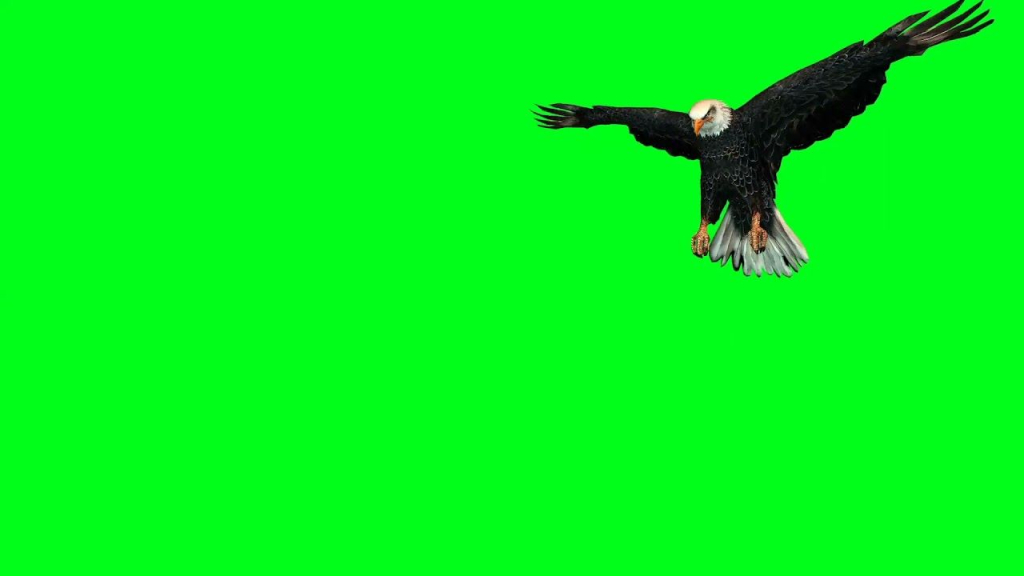Figure 1: Original Green Screen Image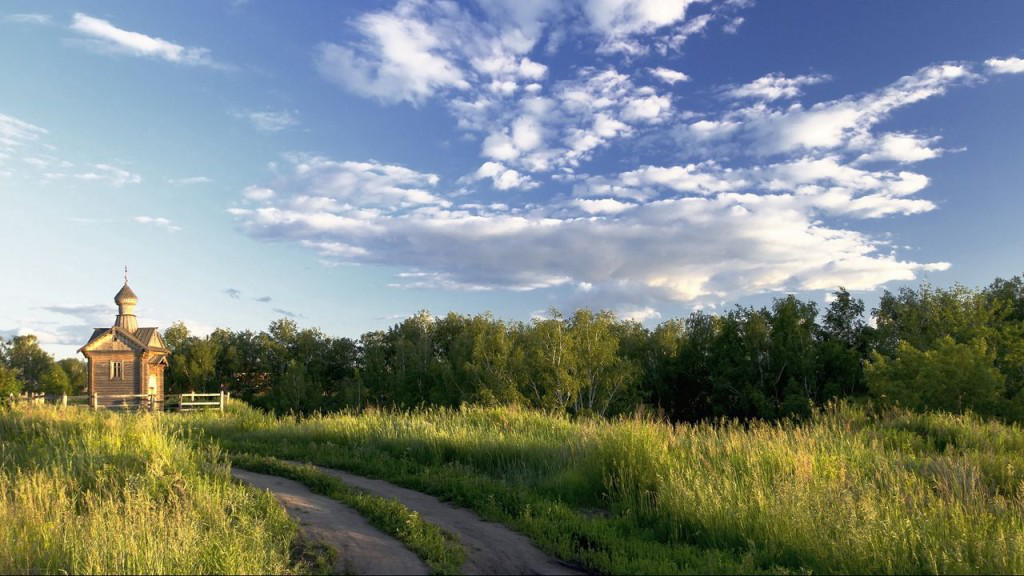Figure 2: Background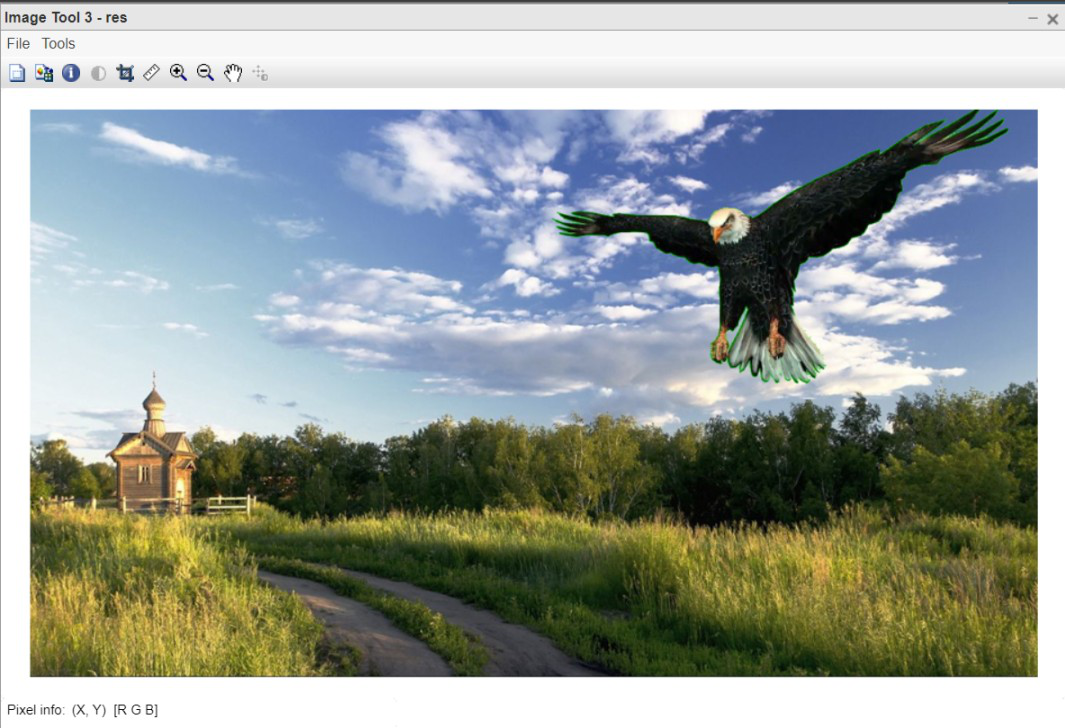Figure 3: Output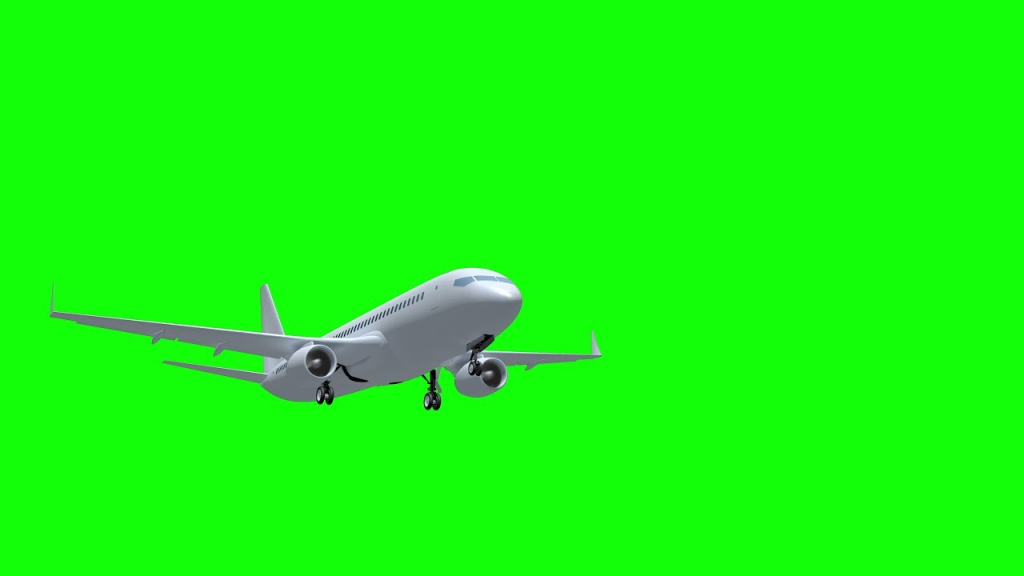Figure 4: Original Green Screen Image

(B)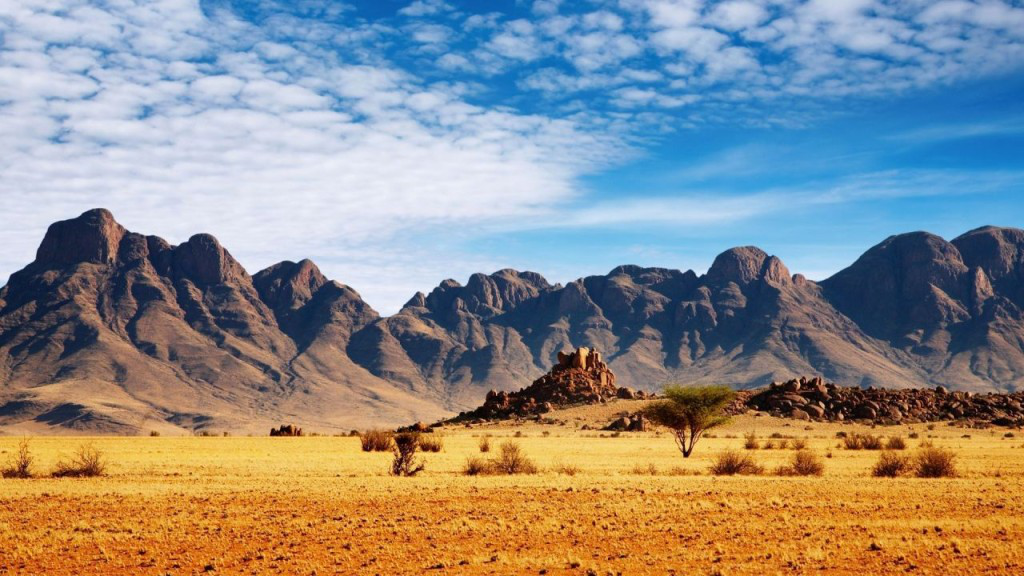Figure 5: Background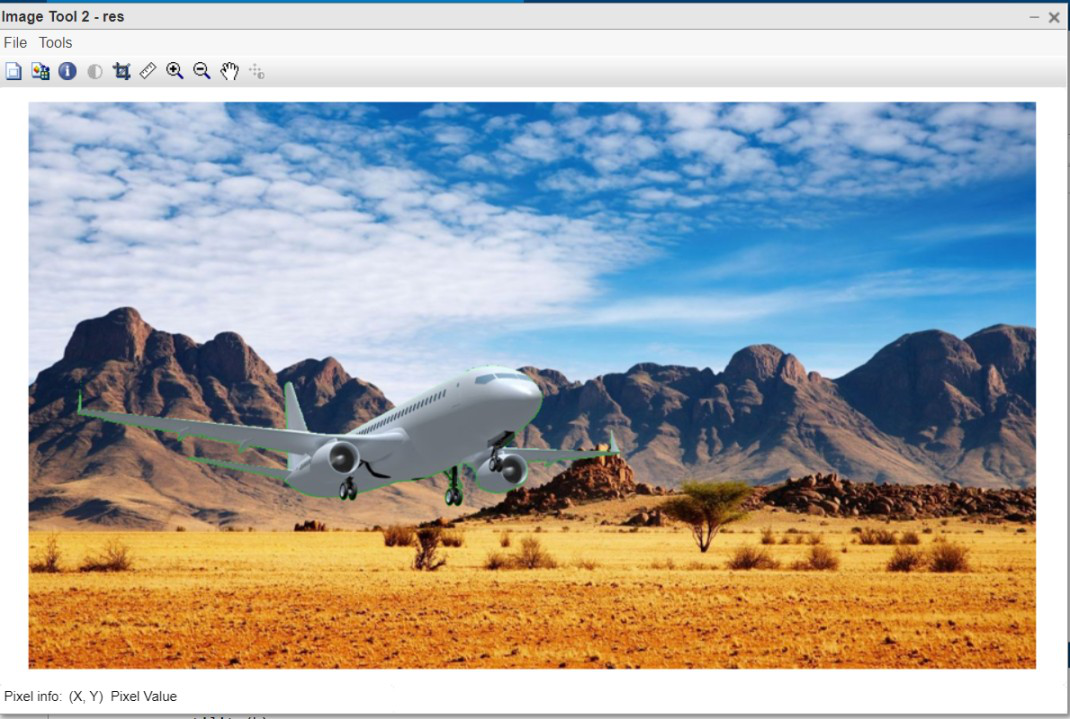Figure 6: Output

Code Explanation:

• function res=GreenScreen(Green_screen_img, Back_ground_img) This line defines the function by passing two image parameters.
• [x,y,~]=size(Green_screen_img); This line returns the size of green screen image.
• res=imresize(img,0.7); This line resizes the original image to 70% width.
• function GreenScreen_Utility(Green_screen_img) This line defines the main function which will carry out the real work.
• backG=[“backG.jpg”,”backG2.jpg”,”backG3.jpg”]; This line defines the list of background images.
• for i=1:length(backG) This line runs the loop over the above defined list of images.
• backGround_img=imread(backG(i)); This line reads one image at a time from the list.
• res=GreenScreen(Green_screen_img,backGround_img); This line calls the Main function by passing 2 image parameters.
• imtool(res,[]); This line displays the resultant image after merging successfully.
• GreenScreen_Utility(k); This line calls the utility function by passing input image.

My Personal Notes arrow_drop_up
Recommended Articles
Page :